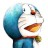# 杰拉斯的博客

## [ACM_POJ_2081]动态规划入门练习（三）Recaman's Sequence杰拉斯 | 时间：2012-04-03, Tue | 5,411 views

### Recaman's Sequence

Time Limit: 3000MS Memory Limit: 60000K
Total Submissions: 17939 Accepted: 7450

#### Description

The Recaman's sequence is defined by a0 = 0 ; for m > 0, am = am−1 − m if the rsulting am is positive and not already in the sequence, otherwise am = am−1 + m.
The first few numbers in the Recaman's Sequence is 0, 1, 3, 6, 2, 7, 13, 20, 12, 21, 11, 22, 10, 23, 9 ...

#### Input

The input consists of several test cases. Each line of the input contains an integer k where 0 <= k <= 500000.
The last line contains an integer −1, which should not be processed.

#### Output

For each k given in the input, print one line containing ak to the output.

```
7
10000
-1
```

```
20
18658
```

#### Source

POJ2081

```#include<stdio.h>
int f;
bool b = {1, 0};	//一个足够大的数组来储存是否已存在
int main(){
f = 0;
for(int i = 1; i < 500001; ++i){
int n = f[i - 1] - i;
if(n < 0 || b[n]){
n = f[i - 1] + i;
}
b[n] = 1;
f[i] = n;
}
int m;
while(scanf("%d", &m) && (m + 1)){
printf("%d\n", f[m]);
}
return 0;
}
```